# Thread: confused relation between pressure and area.

1. ##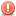confused relation between pressure and area.

As we know, pressure p = force F/ area.pressure decrease with increase in area and increase with decrease in area. now in the nozzle, as area of nozzle decrease pressure reduce and velocity increase. Why?Reply With Quote

2. ##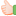Re: confused relation between pressure and area.

See, first you have to differentiate between static fluid and fluid in motion. the nozzles are used in systems of fluids in motion such that the reduction in the area cause the fluid velocity to be increased and therefore the pressure decrease and this is governed by Bernoulli equation. But the relation you are referring govern the static fluid. so how are we rupturing the balloons, it is by squeezing the area at any place of its circular shape (note the fluid in balloon is static) then the pressure of the fluid increases with the area you are reducing of its control volume and after the pressure reaches some value resulted from reducing the area to some value the balloon material couldnt withstand the stress resulting from the fluid pressure and it ruptures. If you note you are actually forming the balloon into a nozzle like shape but again the fluid is static.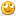ThanksReply With Quote

3. ##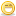Re: confused relation between pressure and area.

Hi pal,as you stated that pressure P=force/area,
here both pressure and area are inversely proportional to each other,so as area decreases the pressure inside the nozzle increases and it will be more than the atm pressure outside the nozzle,therefore the velocity of water is increased as it travels from high pressure to low pressure.Reply With Quote

4. ## Re: confused relation between pressure and area.

hello
As you stated ,
P=F/A
and by continuity equation we know that area of cross section is inversely proportional to velocity of fluid or velocity is directly proportional to the pressure . As a result when area decrease the pressure will increase and in turn it will increase the velocity.Reply With Quote

5. ## Re: confused relation between pressure and area.

Fluid may be Compressible or Incompressible. When we derive the Bernaulli's Equation certain assumption are considered. One of these assumptions is - the fluid is incompressible. And as we know that, as we continuously decrease the back pressure, a nozzle first will behave like a venturimeter, upto this we can assume fluid flow as incompressible flow and we can apply Bernaulli's equation, but further decrease of back pressure will change the flow to compressible flow and now Bernaulli's equation is invalid. All nozzle flow at designed condition is considered as compressible flow and certain different equations are used for study of their behaviour.Reply With Quote

6.

####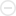Posting Permissions

• You may not post new threads
• You may not post replies
• You may not post attachments
• You may not edit your posts
•